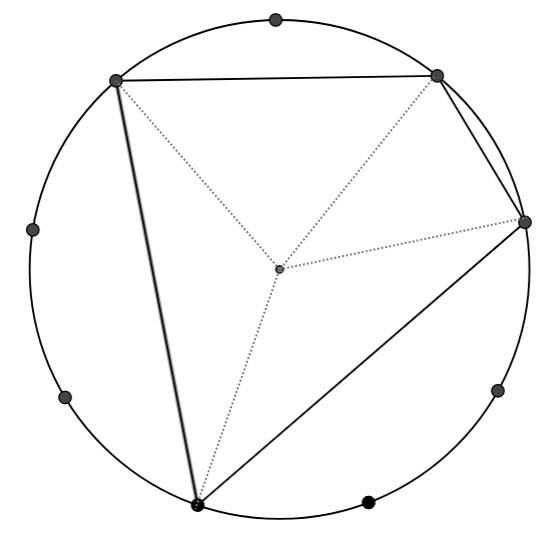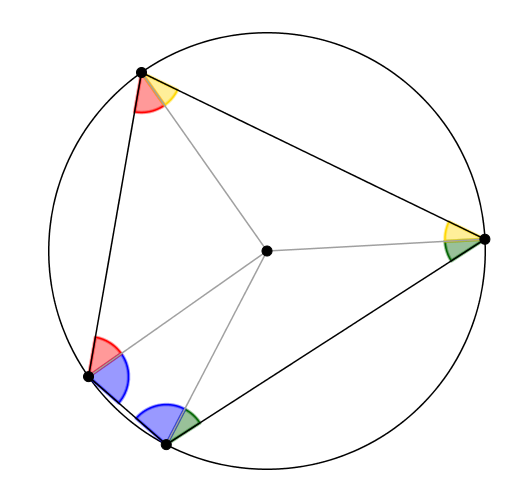#### You may also like### Calendar Capers

Choose any three by three square of dates on a calendar page...Make a set of numbers that use all the digits from 1 to 9, once and once only. Add them up. The result is divisible by 9. Add each of the digits in the new number. What is their sum? Now try some other possibilities for yourself!### Doodles

Draw a 'doodle' - a closed intersecting curve drawn without taking pencil from paper. What can you prove about the intersections?

### Why do this problem?

Working on this problem not only gives students lots of opportunities to practice applying angle rules, but it leads them to an accessible proof that opposite angles in a cyclic quadrilateral sum to $180^\circ$. This task could easily fill two lessons.

### Possible approach

You can print out 9-point Circles for students to work on, or use the GeoGebra applet.

"Draw as many different triangles as you can, by joining the centre dot and any two of the dots on the edge. Can you work out the angles in your triangles, if you know the 9 points are equally spaced around the circle?"

Give students some time to think on their own and then ask them to discuss their ideas with their partners. Then bring the class together and invite students to explain and justify how they calculated each angle.

"We’ve worked out some angles in triangles, now let’s think about quadrilaterals"
"I’d like you to draw some different quadrilaterals by joining four of the nine points around the circle (cyclic quadrilaterals), and then use what you know about angles to work out the angles at the four vertices."
To start with, you may wish students to only draw examples where the centre of the circle lies inside the quadrilateral.

While students are working, you could display an image like the one below to offer a hint for how to work out the angles in the quadrilateral:Once everyone has had a chance to draw some quadrilaterals and work out the angles, bring the class together and ask a few students to read out the four angles going around one of their quadrilaterals, and draw a table on the board to collect the results. It might look something like this:

Angle A Angle B Angle C Angle D
140 60 40 120
100 80 80 100
120 100 60 80
80 140 100 40

“Take a look at the table. What do you notice?”
Give students a little thinking time, drawing attention to pairs of columns A and C, and B and D, if no suggestions are forthcoming.
“It looks as though the opposite angles add up to 180 degrees! I wonder if the same would happen if we used circles with more than 9 points?”

This might be a good place to stop, if you're planning to use this task over several lessons.

Hand out  10 dot, 12 dot, 15 dot and 18 dot circles, and invite different groups of students to draw quadrilaterals, work out the angles, and add their results to the table on the board. Once there is a good deal of evidence, bring the class together again.

“Well we haven’t found any examples where the opposite angles don’t add up to 180 degrees, but that doesn’t mean it will always work – I wonder if we can prove it?”

“Take a look at this diagram and try to come up with a convincing argument that the opposite angles in a cyclic quadrilateral will always add up to 180 degrees.”Give students some time to think about a proof. Circulate and look out for students who notice that:

two yellows + two greens + two blues + two reds = 360 degrees
So, one yellow + one green + one blue + one red = 180 degrees

Opposite angles contain one yellow, one green, one blue and one red,
so opposite angles add up to 180 degrees

Bring the class together to share their thoughts, and if appropriate, write the proof out more formally, using algebra.

### Possible support

Triangles in Circles eases students in a bit more gently to exploring angles on a 9-point circle.

### Possible extension

Students could be challenged to think about cyclic quadrilaterals where the centre of the circle is not contained within the quadrilateral.

Other circle theorems could be explored in a similar way - see Subtended Angles and Right Angles.